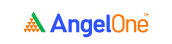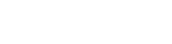# Pair trading jargon and terms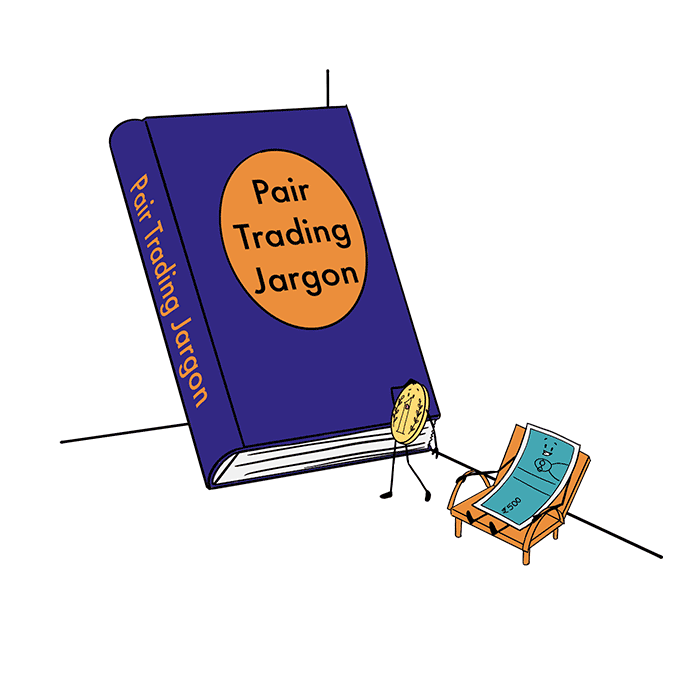Pair trading is a strategy that requires some degree of market expertise. Getting a grip on the practical aspects of pair trading is important. And so is understanding the theory involved. And step one of making sense of all the academic and statistical ideas behind pair trading is to decode the trading jargon surrounding this concept.

And that’s where this chapter comes in. Before you move on to learning about making a pair trade, you’ll need to understand some of the key trading terms involved. Let’s look at some such words and phrases.

### Correlation

Recall the definition of pair trading from the earlier chapter? It’s all about picking correlated stocks, right? But what is this correlation, anyway?

Mathematically (or statistically) speaking, correlation is a concept that tells you how closely two given variables are related, if at all they are. It is measured and expressed using the correlation coefficient. The correlation coefficient ranges from -1 to +1. Wondering what the plus and minus signs mean? Let’s break down the correlation coefficient into its two components to understand its significance better.

• The symbol tells you the direction of movement. A positive symbol means that the two variables tend to move in the same direction, while a negative symbol means they typically move in opposite directions.
• The number following the symbol tells you the strength of the correlation. A correlation coefficient of 0.90, for instance, is stronger than a correlation coefficient of 0.60.

So, what does a correlation coefficient of zero suggest? Well, it means that there is zero correlation between the two variables.

That clears up the statistical and conceptual part of this trading jargon. Now, let’s apply the concept of correlation to stock prices to understand how it is applied. Take the same two companies that we discussed in the previous chapter - TCS and Infosys. Say they have a correlation coefficient of +0.94. Here’s what that means.

• All other things remaining constant, if the price of TCS shares moves up by, say 1.2%, it means that the price of Infosys shares will also likely move up.
• This is because the two stocks have a positive correlation - as well as a high correlation coefficient.
• But, you will not be able to predict the extent by which Infosys shares will rise.

And, here’s another thing about correlation. On its own, it may be a purely statistical concept. However, if you wish to identify pair trading opportunities using correlation, you’ll need to look for a logical reason behind a potentially high correlation coefficient. What this means is, just because two stocks exhibit high levels of correlation, it does not mean they’re good candidates for pair trading. Beyond the numerical correlation, there needs to be some logical and intuitive reason for the link between the two stocks.

Here’s a quick couple of examples to explain this idea better.

• Like we saw earlier, if TCS and Infosys stocks show a correlation coefficient of 0.94, they may make good candidates for a pair trade.
• On the other hand, let’s take two other stocks - Hindustan Unilever and HDFC Bank. Now, let’s say the correlation coefficient for these stocks is +0.82. Now, that’s a reasonably high correlation coefficient. But does it make Hindustan Unilever and the HDFC Bank shares ideal for a pair trade.

No , it does not. That’s because HDFC Bank belongs to the banking sector, while Hindustan Unilever is an FMCG company, and the entities do not have any logical or intuitive connection.

### Differential

You know what correlation is now. But how do you establish a correlation between two stocks? To do that, there are different factors that traders and analysts consider. One such factor is the differential.

It’s essentially the difference in the closing price of the stocks in consideration. Here’s the formula for the differential.

 Differential = Closing price of stock A - Closing price of stock B (or vice versa)

Let’s take up the closing prices of TCS and Infosys shares for a brief period and understand how to calculate the differential.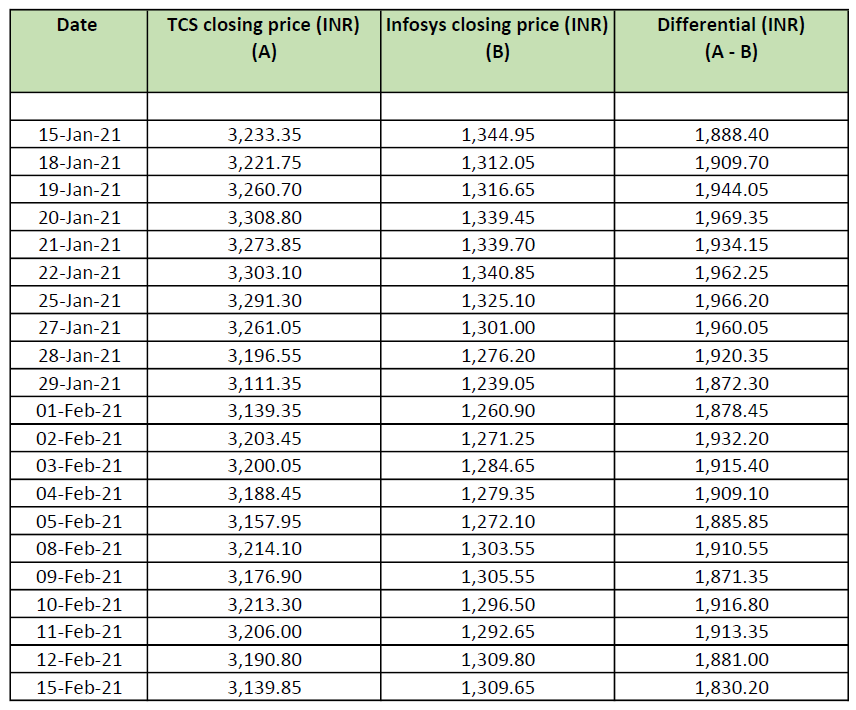Now, the spread is another metric that can be used to figure out the correlation between two stocks. To calculate the spread, you’ll first need to calculate the difference in the previous day’s closing price and the present day’s closing price for each stock, over the period you’re considering. This gives you the change in closing price of each stock on a day-to-day basis.

Then, you’ll have to compute the difference between the daily changes in closing prices of the two stocks.

The formula should make it clearer.

 Spread = Daily change in closing price of stock A - Daily change in closing price of stock B (or vice versa)

Let’s take up the closing prices of TCS and Infosys shares for the same period considered above and understand how to calculate the spread.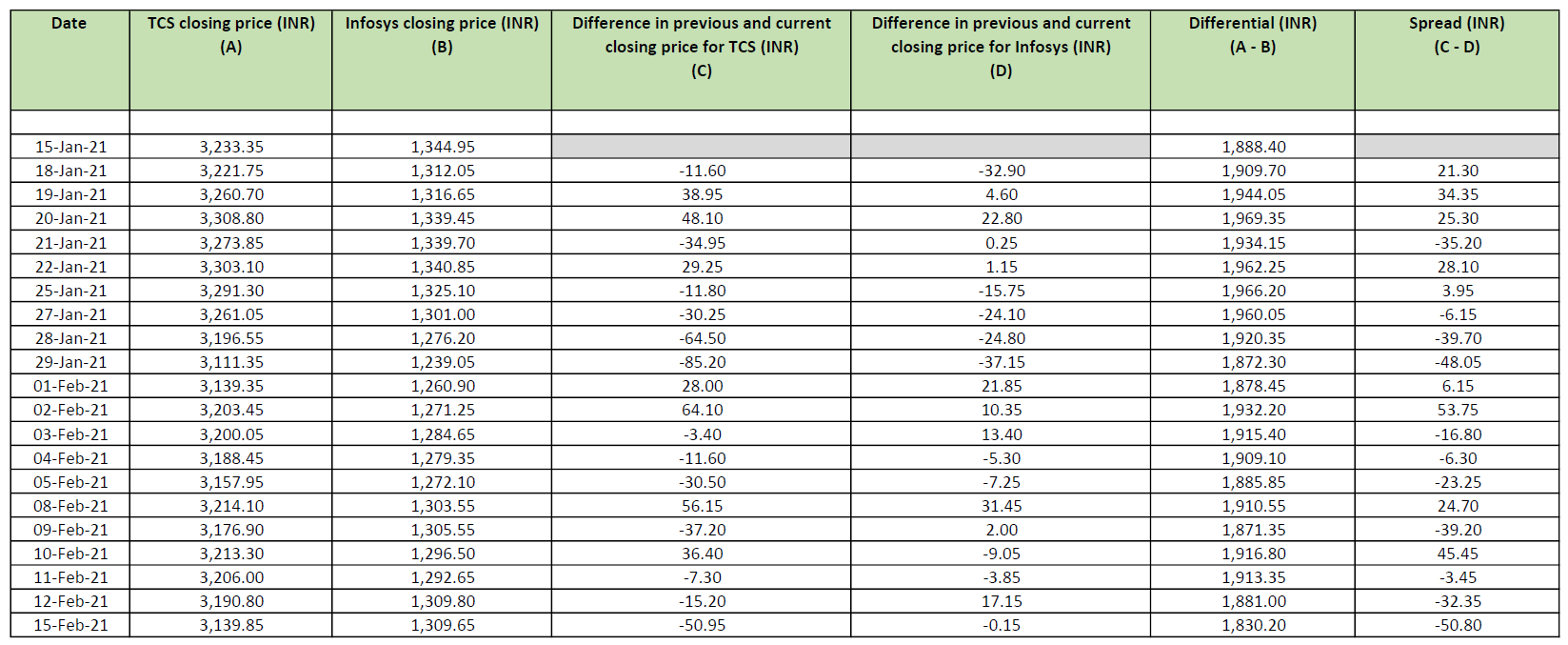### Price ratio

This one’s pretty straightforward. It’s calculated as the ratio of the closing price of stock A to the closing price of stock B (or vice versa).

Check out the formula below.

 Price ratio = Closing price of stock A ÷ Closing price of stock B (or vice versa)

And now, you know the drill. Let’s take up the closing prices of TCS and Infosys shares for the same period considered above and check out the price ratios.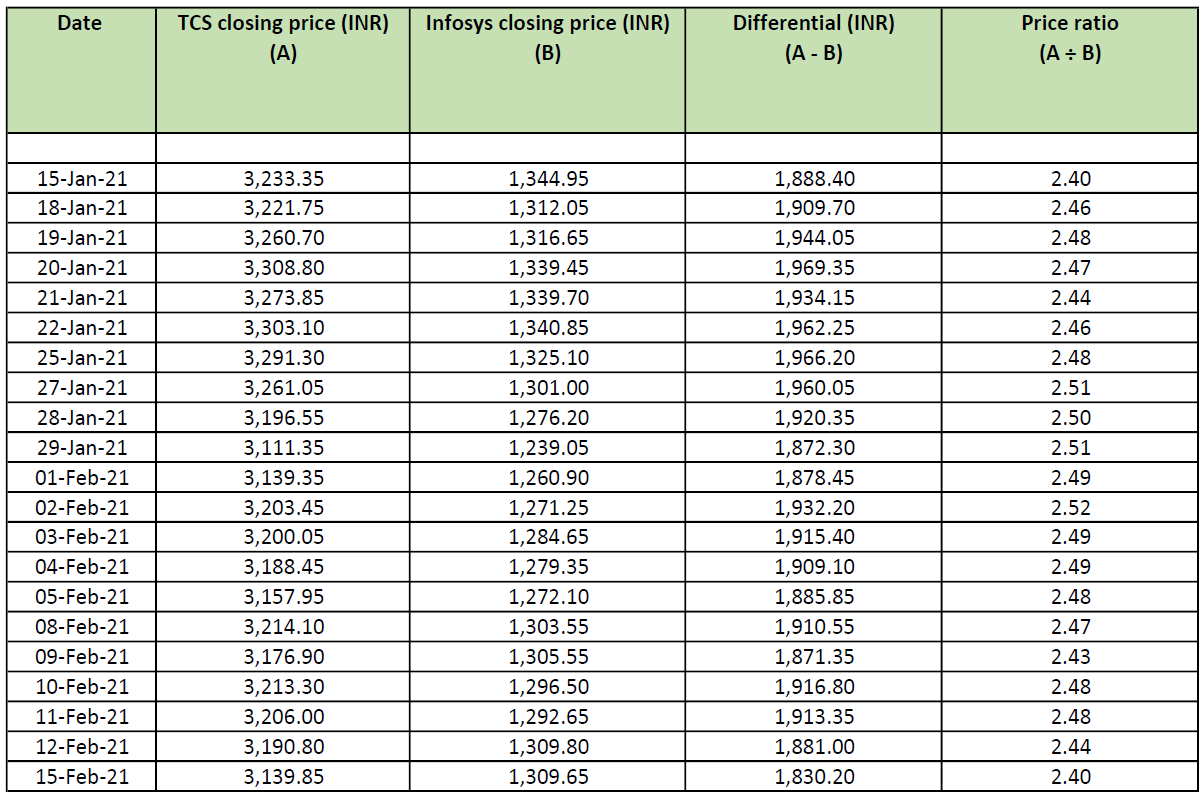So, you’ve seen three variables that are generally used to identify the relationship or the correlation between two stocks. Let’s plot them on a line graph and see how it turns out.

1. The differential graph: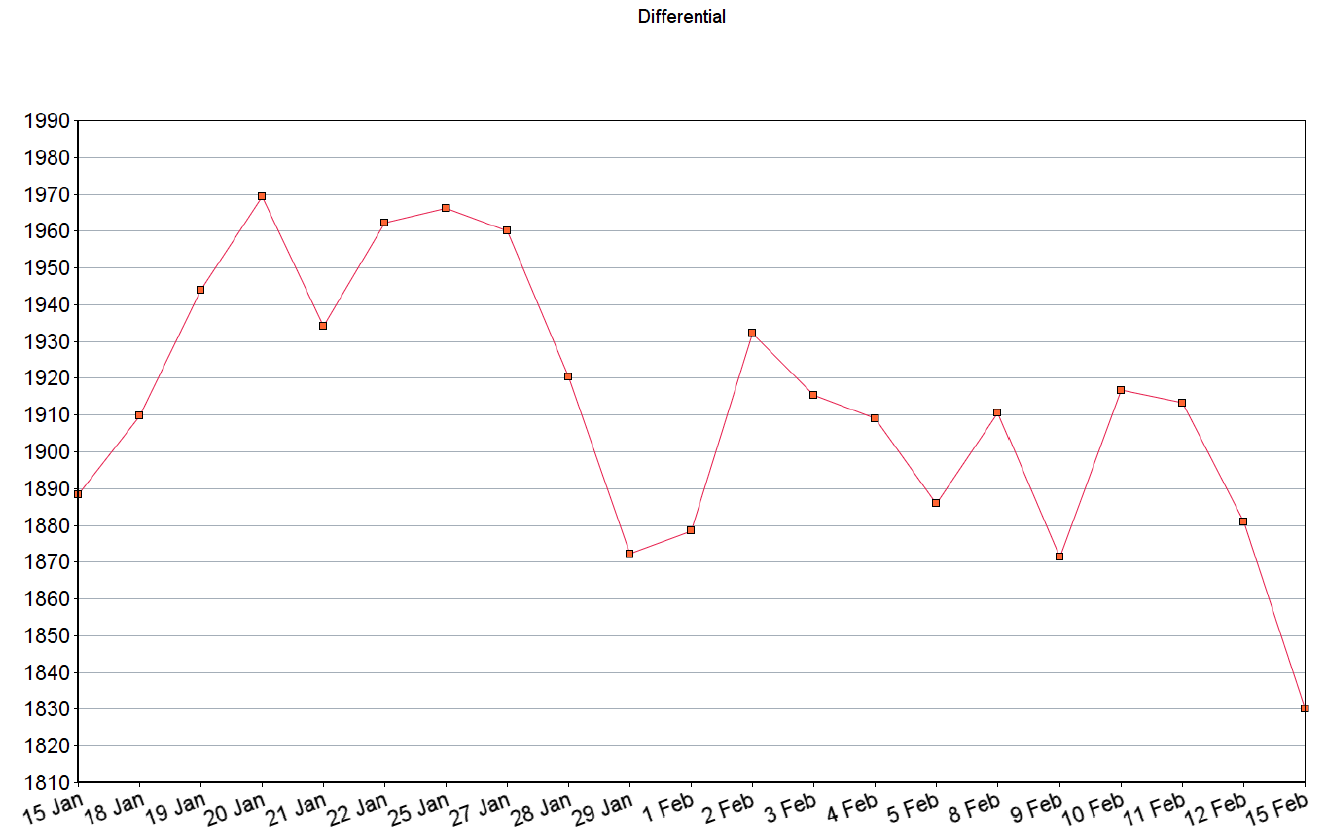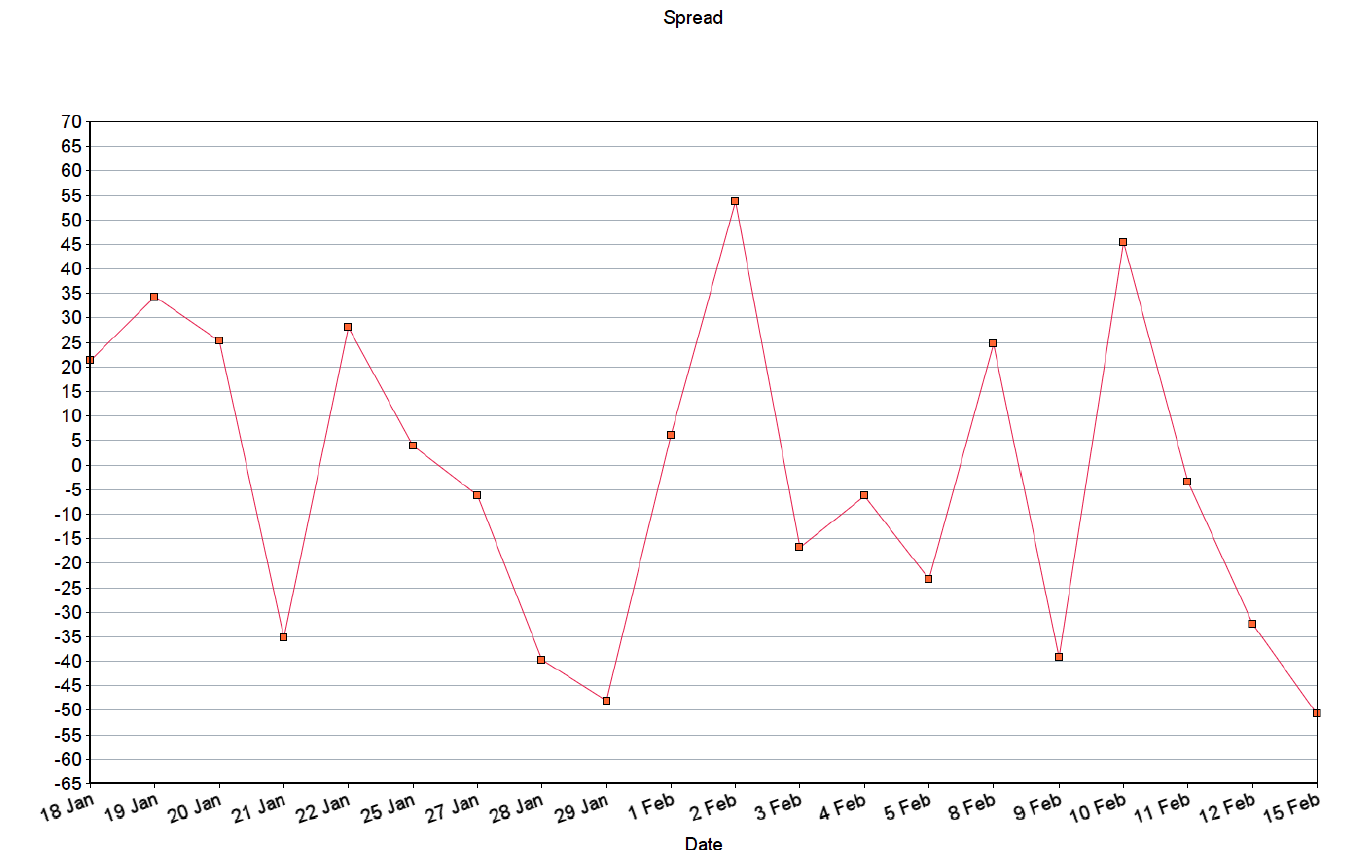3. The price ratio graph: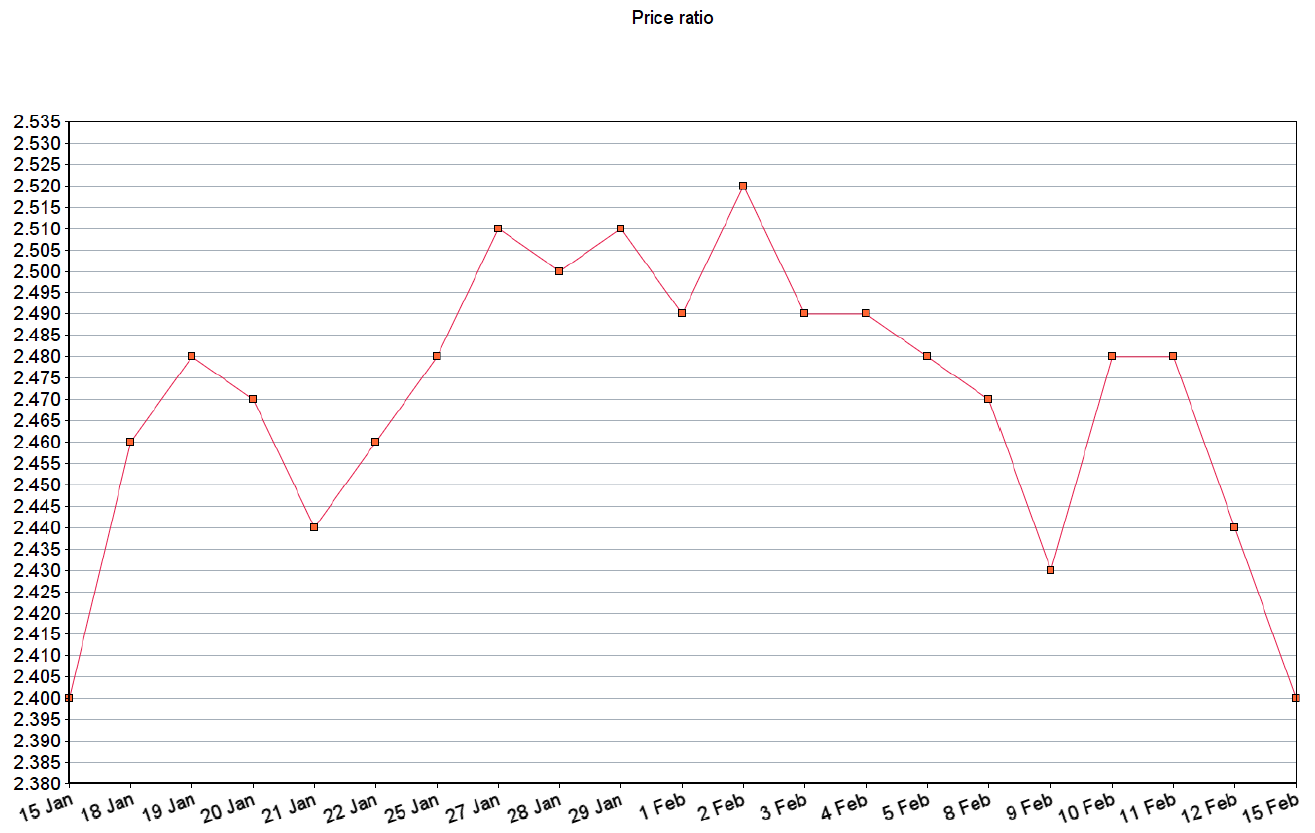### Divergence

The movement of the variables gives you some insight into how the stock prices are behaving. For example, take the price ratio. The ratio may increase in any of the following cases:

• If the price of stock A increases, but the price of stock B remains nearly constant
• If the price of stock B decreases steeply, but the price of stock A remains nearly constant

Here, we’re assuming that we’re calculating the ratio with stock A’s price as the numerator and stock B’s price as the denominator.

As you may have guessed, in both the cases described above, the correlative behavior between the two stocks is temporarily weakened because they’re moving apart. That’s when a divergence occurs, leading to an increased spread between the two stocks, and thereby, an increased ratio. The graph typically moves upward during such points, giving traders an indication that a divergence is occurring.

A divergence trade is initiated when a divergence has been spotted in the prices of two correlated stocks.

### Convergence

Convergence, evidently, is the opposite of divergence. Let’s take up the ratio again, and see how it reacts during a convergence. Typically, the ratio decreases due to any of the following possible reasons:

• The price of stock A decreases, while that of stock B increases or remains nearly constant
• The price of stock B increases, while that of stock A decreases or remains nearly constant

Again, here, we’re assuming that we’re calculating the ratio with stock A’s price as the numerator and stock B’s price as the denominator.

In the two cases described above, the correlative behavior between the two stocks is temporarily weakened because they’re moving closer. That’s when a convergence occurs, leading to a decreased spread between the two stocks, and consequently, a decreased ratio. The graph typically moves downward during such points, giving traders an indication that a convergence is occurring.

A convergence trade is initiated when a convergence has been spotted in the prices of two correlated stocks.

#### Wrapping up

That clears up some of the trading jargon that you’ll typically come across when you’re discussing pair trading - particularly the correlation method. Now that that’s done, it’s time to revisit some high school math and recap some essential statistical tools. Ready? Head to the next chapter to learn more.

#### A quick recap

• Correlation tells you how closely two given variables are related, if at all they are.
• It is measured and expressed using the correlation coefficient. The correlation coefficient ranges from -1 to +1.
• Just because two stocks exhibit high levels of correlation, it does not mean they’re good candidates for pair trading. Beyond the numerical correlation, there needs to be some logical and intuitive reason for the link between the two stocks.
• The differential is the difference in the closing price of the stocks in consideration.
• The spread is the difference between the daily changes in closing prices of the two stocks.
• The price ratio is calculated as the ratio of the closing price of stock A to the closing price of stock B (or vice versa).
• Divergence occurs when the correlative behavior between the two stocks is temporarily weakened because they’re moving apart.
• Convergence occurs when the correlative behavior between the two stocks is temporarily weakened because they’re moving closer.Take the quiz for this chapter & mark it complete.

How would you rate this chapter?

Get Information Mindfulness!

Catch-up With Market

News in 60 Seconds.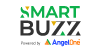The perfect starter to begin and stay tuned with your learning journey anytime and anywhere.